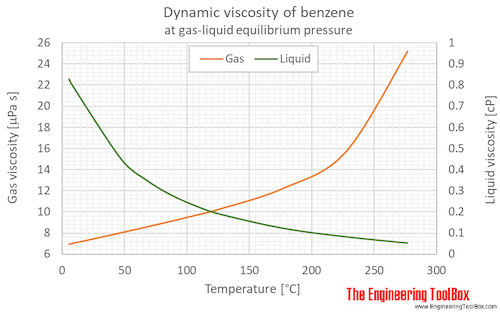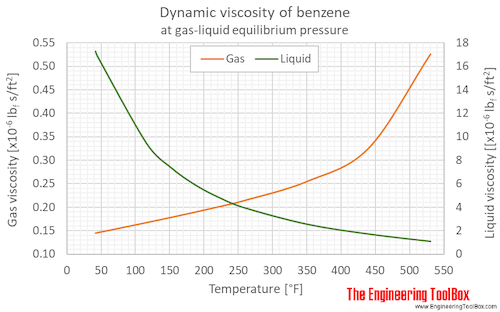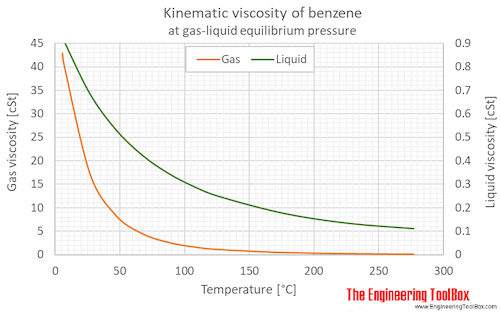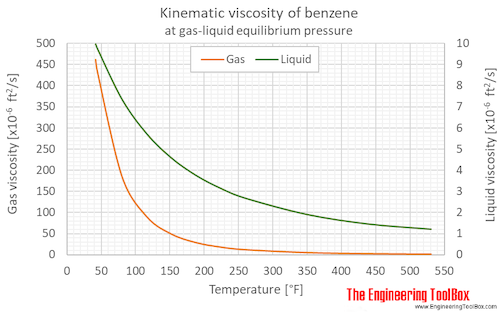Engineering ToolBox - Resources, Tools and Basic Information for Engineering and Design of Technical Applications!

# Benzene - Dynamic and Kinematic Viscosity

## Online calculator, figures and table showing dynamic and kinematic viscosity of benzene, C6H6, at varying temperature and pressure - Imperial and SI Units

The viscosity of a fluid is a measure of its resistance to gradual deformation by shear stress or tensile stress.

For further definitions, go to Absolute (dynamic) and kinematic viscosityAbsolute or dynamic viscosity is used to calculate Reynold's Number to determine if a fluid flow is laminar, transient or turbulent.

Tabulated values and viscosity units conversion are given below the figures.

Benzene phase diagram

### Online Liquid Benzene Viscosity Calculator

The calculator below can be used to estimate liquid benzene dynamic or kinematic viscosity at given temperatures. The calculator gives the viscosity at saturation pressure. For most practicle issues this can be used for liquid benzene at any pressure up to the critical point (289°C, 49 bara or 552°F, 712 psia)
The output dynamic viscosity is given as Pa*s, N*s/m2, cP, mPa*s, lbf*s/ft2 and lbm/(ft*h),
while the kinematic viscosity is given as cSt, m2/s, and ft2/s

Temperature

Choose the actual unit of temperature:

°C  °F  °R

See also other properties of Benzene at varying temperature and pressure:  Density and specific weight, and Thermophysical properties at standard conditions,
as well as dynamic and kinematic viscosity of propane and water.

Dynamic viscosity of benzene at gas-liquid equilibrium pressure:Back to top
Kinematic viscosity of benzene at gas-liquid equilibrium pressure:Dynamic (absolute) and kinematic viscosity of benzene at given temperatures and pressures, SI and Imperial units:

For full table Dynamic and Kinematic Viscosity - rotate the screen!

 State Temperature Pressure Dynamic (Absolute) Viscosity Kinematic viscosity [K] [°C] [°F] [bara] [psia] [μPa s] [cP] [lbf s/ft2 *10-6] [lbm/ft s*10-6] [lbm/ft h] [cSt], [m2/s*10-6] [ft2/s*10-6] Liquid at equilibrium 278.70 5.55 41.99 0.0480 0.696 828.1 0.8281 17.30 556.5 2.003 0.9266 9.974 280 6.9 44.3 0.0515 0.747 810.4 0.8104 16.93 544.6 1.960 0.9082 9.775 300 26.9 80.3 0.138 2.00 596.2 0.5962 12.45 400.6 1.442 0.6841 7.364 320 46.9 116 0.321 4.65 452.5 0.4525 9.451 304.1 1.095 0.5324 5.730 340 66.9 152 0.661 9.59 352.7 0.3527 7.366 237.0 0.8532 0.4258 4.583 360 86.9 188 1.24 18.0 281.1 0.2811 5.871 188.9 0.6800 0.3488 3.755 380 107 224 2.16 31.3 228.4 0.2284 4.770 153.5 0.5525 0.2918 3.141 400 127 260 3.53 51.2 188.5 0.1885 3.937 126.7 0.4560 0.2485 2.674 450 177 350 9.72 141 122.5 0.1225 2.558 82.32 0.2963 0.1768 1.904 500 227 440 21.7 314 82.34 0.08234 1.720 55.33 0.1992 0.1349 1.452 550 277 530 42.1 611 52.37 0.05237 1.094 35.19 0.1267 0.1124 1.210 Gas at equilibrium 278.7 5.6 42.0 0.0480 0.696 6.960 0.00696 0.1454 4.677 0.01684 42.88 461.6 280 6.9 44.3 0.051 0.747 6.994 0.006994 0.1461 4.700 0.01692 40.36 434.4 300 26.9 80.3 0.138 2.00 7.509 0.007509 0.1568 5.046 0.01816 17.22 185.3 320 46.9 116 0.321 4.65 8.031 0.008031 0.1677 5.397 0.01943 8.404 90.46 340 66.9 152 0.661 9.59 8.564 0.008564 0.1789 5.755 0.02072 4.574 49.24 360 86.9 188 1.24 18.0 9.113 0.009113 0.1903 6.124 0.02205 2.699 29.06 380 107 224 2.16 31.3 9.685 0.009685 0.2023 6.508 0.02343 1.708 18.38 400 127 260 3.53 51.2 10.3 0.0103 0.2151 6.921 0.02492 1.144 12.31 450 177 350 9.72 141 12.22 0.01222 0.2552 8.211 0.02956 0.5029 5.413 500 227 440 21.7 314 15.62 0.01562 0.3262 10.50 0.03779 0.2711 2.918 550 277 530 42.1 611 25.18 0.02518 0.5259 16.92 0.06091 0.1675 1.803 Liquid 298 25 77 1 14.5 604 0.604 12.6 406 1.46 0.691 7.44 323 50 122 1 14.5 436 0.436 9.11 293 1.05 0.515 5.54 348 75 167 1 14.5 335 0.335 7.00 225 0.810 0.409 4.40

Unit conversion:

Viscosity, Dynamic (Absolute)

Absolute or Dynamic Viscosity Unit Converter
centipoise [cP], gram/(centimeter second) [g/(cm s)] = poise [P],  kilogram/meter second [kg/m s]=newton second/square meter [N s/m2]= pascal second [Pa s], pound/(foot hour) [lb/(ft h)], pound/(foot second) [lb/(ft s)], reyn [reyn]

• 1 cP = 0.001 Pa s = 0.01 P = = 0.01 g/(cm sec) = 6.72197x10-4 lb/(ft s) = 2.4191 lb/(ft h)
• 1 lb/(ft h) = 0.00027778 lb/(ft s) = 0.00041338 Pa s = 0.0041338 P = 0.41338 cP
• 1 lb/(ft s) = 3600 lb/(ft h) = 1.48816 Pa s = 14.8816 P = 1488.16 cP
• 1 kg/(m s ) = 1 (N s)/m2 = 1 Pa s = 10 P = 1000 cP = 0.672197 lb/(ft s) = 2419.09 lb/(ft h)
• 1 (N s)/m2 = 1 kg/(m s ) = 1 Pa s = 10 P = 1000 cP = 0.672197 lb/(ft s) = 2419.09 lb/(ft h)
• 1 P = 1 g/(cm s) = 0.1 Pa s = 100 cP = 0.067197 lb/(ft s) = 241.909 lb/(ft h)
• 1 Pa s = 1 kg/(m s) = 1 (N s)/m2 = 10 P = 1000 cP = 0.672197 lb/(ft s) = 2419.08 lb/(ft h) =  0.00014504 reyn
• 1 reyn = 6894.76 Pa s

Viscosity, Kinematic

Kinematic Viscosity Unit Converter
centistoke [cSt] = square millimeter/second [mm2/s], square foot/hour [ft2/h], square foot/second [ft2/s], square inch/second [in2/s], square meter/hour [m2/h], square meter/second [m2/s], stoke [St] = square centimeter/second [cm2/s]

• 1 cm2/s = 1 St = 100 mm2/s = 100 cSt = 1x10-4 m2/s = 0.36 m2/h = 1.07639x10-3 ft2/s = 3.875008 ft2/h = 0.1550003 in2/s
• 1 cSt = 1 mm2/s = 0.01 St = 1x10-6 m2/s = 0.0036 m2/h = 1.07639x10-5 ft2/s = 0.03875008 ft2/h = 0.001550003 in2/s
• 1 ft2/h = 2.7778x10-4 ft2/s = 0.04 in2/s = 2.58064x10-5 m2/s = 0.09290304 m2/h = 25.8064 cS = 0.258064 St
• 1 ft2/s = 3600 ft2/h = 144 in2/s = 0.09290304 m2/s = 334.451 m2/h = 92903.04 cSt = 929.0304 St
• 1 in2/s = 0.0069444 ft2/s = 25 ft2/h = 0.00064516 m2/s = 2.322576 m2/h = 645.16 cSt = 6.4516 St
• 1 m2/h = 1/3600 m2/s = 2.7778x10-4 m2/s = 2.7778 cm2/s = 277.78 mm2/s = 277.78 cSt = 2.7778 St = 0.00298998 ft2/s = 10.7639 ft2/h = 0.430556 in2/s
• 1 m2/s = 3600 m2/h = 1x104 cm2/s = 1x104 St = 1x106 mm2/s = 1x106 cSt = 10.7639 ft2/s = 38750.08 ft2/h = 1550003 in2/s
• 1 mm2/s = 1 cSt = 1x10-6 m2/s = 0.0036 m2/h = 0.01 cm2/s = 0.01 St = 1.07639x10-5 ft2/s = 0.03875008 ft2/h = 0.001550003 in2/s
• 1 St = 1 cm2/s = 100 cSt = 100 mm2/s = 1x10-4 m2/s = 0.36 m2/h = 1.076x10-3 ft2/s = 3.875008 ft2/h = 0.1550003 in2/s

## Related Topics

• Fluid Mechanics - The study of fluids - liquids and gases. Involves velocity, pressure, density and temperature as functions of space and time
• Material Properties - Material properties for gases, fluids and solids - densities, specific heats, viscosities and more
• Thermodynamics - Effects of work, heat and energy on systems
• Viscosity - Documents giving viscosity of different kind of chemical species at varying conditions

## Tag Search

• en: benzene benzol dynamic kinematic viscosity temperature

## Engineering ToolBox - SketchUp Extension - Online 3D modeling!

Add standard and customized parametric components - like flange beams, lumbers, piping, stairs and more - to your Sketchup model with the Engineering ToolBox - SketchUp Extension - enabled for use with the amazing, fun and free SketchUp Make and SketchUp Pro .Add the Engineering ToolBox extension to your SketchUp from the SketchUp Pro Sketchup Extension Warehouse!

## Privacy

We don't collect information from our users. Only emails and answers are saved in our archive. Cookies are only used in the browser to improve user experience.

Some of our calculators and applications let you save application data to your local computer. These applications will - due to browser restrictions - send data between your browser and our server. We don't save this data.

## Citation

• Engineering ToolBox, (2018). Benzene - Dynamic and Kinematic Viscosity. [online] Available at: https://www.engineeringtoolbox.com/benzene-benzol-dynamic-kinematic-viscosity-temperature-d_2073.html [Accessed Day Mo. Year].

Modify access date.

. .

#### Scientific Online Calculator11 8